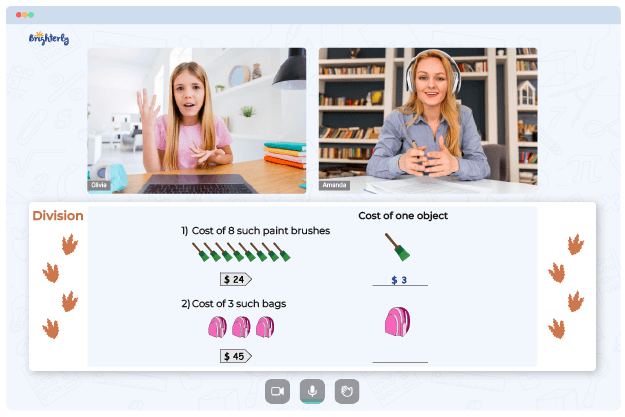# Long Division Worksheets Grade 6

Long division is a mathematical method used for dividing two numbers. In grade 6, students typically learn long division with two-digit divisors and four-digit dividends. Our 6th grade long division worksheets will help students in grade 6 to understand and solve problems related to long division. The long division worksheets grade 6 will include practice problems that involve dividing a four-digit number by a two-digit number.

This long division 6th grade worksheet starts with simple problems where the divisor and dividend are easy to divide and gradually increase in difficulty as students progress. The long division worksheet grade 6 will also include word problems that require students to use long division to solve real-world situations.

Math for Kids

Is Your Child Struggling With Math?
1:1 Online Math Tutoring## Benefits of Long Division Grade 6 Worksheet

Long division is an essential math skill for students in grade 6, and it has several benefits:

• Improving problem-solving skills: Long division requires students to break down complex problems into smaller, more manageable steps. This process helps them develop their problem-solving skills, which can be applied to other areas of life.
• Strengthening computational skills: Long division helps students improve their computational skills, including their ability to add, subtract, multiply, and divide large numbers accurately.
• Enhancing critical thinking skills: Long division requires students to analyze problems, think critically, and make decisions based on the information they have. These skills are essential for success in all areas of life.### Long Division Worksheets Grade 6 PDF### Long Division Worksheets Grade 6 PDF

Long Division Worksheets Grade 6 PDF### Long Division Worksheets Grade 6 PDF### Long Division Worksheets Grade 6 PDF

Troubles with Division?• Does your child struggle to understand division lessons?
• Try studying with an online tutor.

Is your child having trouble grasping of division? An online tutor could provide the necessary guidance.